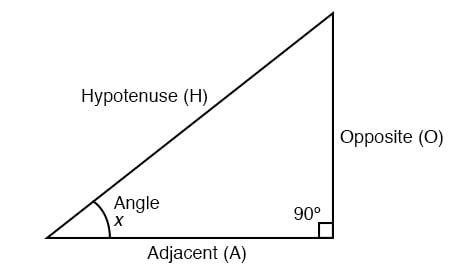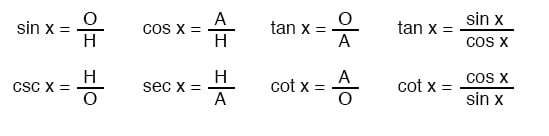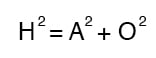Vol. EE Reference
Chapter 5 Trigonometry Reference

# Right Triangle TrigonometryA right triangle is defined as having one angle precisely equal to 90o (a right angle).

### Trigonometric IdentitiesH is the Hypotenuse, always being opposite the right angle. Relative to angle x, O is the Opposite and A is the Adjacent.

“Arc” functions such as “arcsin”, “arccos”, and “arctan” are the complements of normal trigonometric functions.

These functions return an angle for a ratio input. For example, if the tangent of 45o is equal to 1, then the “arctangent” (arctan) of 1 is 45o.

“Arc” functions are useful for finding angles in a right triangle if the side lengths are known.

### The Pythagorean TheoremRELATED WORKSHEETS:

#### Lessons in Electric Circuits

##### Volumes »
Published under the terms and conditions of the Design Science License